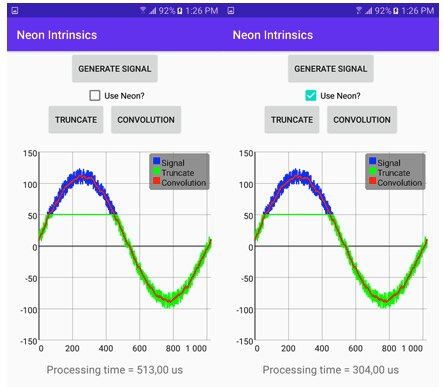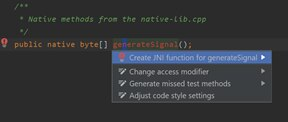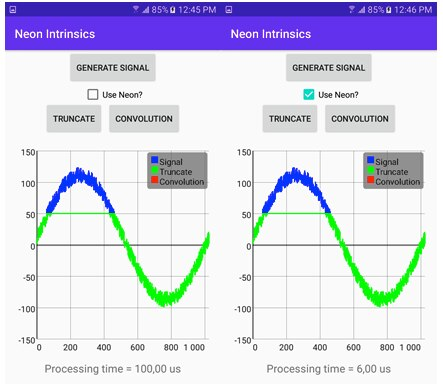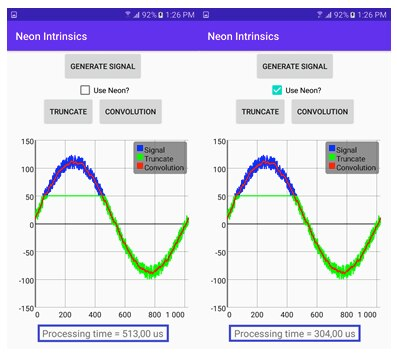## Overview

In this guide, we show you how to write efficient code that can be used for signal and image processing, neural networks, or game applications.

We develop an Android app that uses native C++ code to perform signal processing. While we do this, we show how easily you can combine Java with native code to perform computation-intensive work.

Single Instruction, Multiple Data (SIMD) architectures allow you to parallelize code execution. SIMD allows you to perform the same operation on an entire sequence, or vector, of data during one instruction. This means that you can use SIMD to significantly improve the performance of your Android mobile or IoT apps.

Processor manufacturers provide tools to make SIMD optimizations easily accessible for developers. Arm Neon intrinsics are built-in functions that you can access from C/C++ code. The compiler replaces these functions with assembly instructions that closely map C/C++ code. As demonstrated in this guide, you can use Neon intrinsics to easily vectorize your code with just a few changes.

We will create an Android app that uses Neon intrinsics to process a 1D signal. The signal is the sine wave with added random noise. It shows how to implement the truncate thresholding and convolution of that signal. Thresholding is usually the first step in various image-processing algorithms, while convolution is the primary signal and image-processing tool.

At the end of this guide, you can Check your knowledge. You will know how to use Neon intrinsics for Arm-powered mobile devices.

## Before you begin

There are a few things that you need to be familiar with before you work through this guide.

We discuss truncation and convolution in this guide. If you are not familiar with these concepts, here is a short introduction:

Truncate: To truncate something is to shorten it or cut part of it off. In computer science, the term is often used about data types or variables, like floating-point numbers and strings. For example, a function may truncate the decimal portion of a floating-point number to make it an integer.

Convolution: In computer science, specifically formal languages, convolution is a function which maps a tuple of sequences into a sequence of tuples. Convolution is sometimes called zip. The name zip comes from the action of a zipper, which interleaves two formerly separate sequences.

Android Studio is used for thresholding, and the code is tested on a Samsung SM-J710F phone. The full source code is available from the GitHub repository.

We also recommend that you read our guide Getting started with Neon Intrinsics on Android before reading this guide. In that guide it showed how to set up Android Studio to use Arm Neon intrinsics for Android applications.

The following diagram is what you should see in your set up for truncating and convolution in Android Studio:## The application structure

In this section of the guide, we work through the steps in building out the application structure with code examples. A key part of app development is the application structure. The following steps take you through the application structure:

1. Declare the UI of the application by modifying `activity_main.xml`, which is under `app/src/main/res/layout.`
2. As shown in the following code, use one linear layout to align the controls vertically. The additional linear layout is used here to position the Truncate and Convolution buttons next to each other. The `ScrollView` wraps all controls. This means that the user can scroll down if the screen is too small to display everything at once:

`<?xml version="1.0" encoding="utf-8"?><ScrollView xmlns:android="http://schemas.android.com/apk/res/android"        android:layout_width="match_parent"    android:layout_height="wrap_content"><LinearLayout xmlns:android="http://schemas.android.com/apk/res/android"        xmlns:tools="http://schemas.android.com/tools"        android:layout_width="match_parent"        android:layout_height="match_parent"        android:orientation="vertical"        tools:context=".MainActivity">    	<Button            android:id="@+id/buttonGenerateSignal"            android:layout_width="wrap_content"            android:layout_height="wrap_content"            android:layout_gravity="center_horizontal"            android:onClick="buttonGenerateSignalClicked"            android:text="Generate Signal"            android:layout_margin="5dp"/>    	<CheckBox            android:id="@+id/checkboxUseNeon"            android:layout_width="wrap_content"            android:layout_height="wrap_content"            android:layout_gravity="center_horizontal"            android:text="Use Neon?" />    	<LinearLayout            android:layout_width="wrap_content"            android:layout_height="wrap_content"            android:layout_gravity="center_horizontal">            <Button                android:id="@+id/buttonTruncate"                android:layout_width="wrap_content"                android:layout_height="wrap_content"                android:layout_gravity="center_horizontal"                android:onClick="buttonTruncateClicked"                android:text="Truncate"                android:layout_margin="5dp"/>            <Button                android:id="@+id/buttonConvolution"                android:layout_width="wrap_content"                android:layout_height="wrap_content"                android:layout_gravity="center_horizontal"                android:onClick="buttonConvolutionClicked"                android:text="Convolution"                android:layout_margin="5dp"/>        </LinearLayout>        <com.jjoe64.graphview.GraphView            android:id="@+id/graph"            android:layout_width="match_parent"            android:layout_height="350dp"            android:layout_margin="10dp"/>    	<TextView            android:id="@+id/textViewProcessingTime"            android:layout_width="wrap_content"            android:layout_height="wrap_content"            android:layout_gravity="center_horizontal"            android:textSize="18sp" />    </LinearLayout></ScrollView>`
3. To plot the signal before and after processing, use the GraphView library. Install this library by including the following line under the dependency node of your `build.gradle`:

`implementation 'com.jjoe64:graphview:4.2.2'`

4. Then, include the `GraphView` in the UI by using the <com.jjoe64.graphview.GraphView> attribute. When `GraphView` is included, you get a reference to the GraphView instance like you would get with any other Android control.

In the `configureGraph` method that we invoke under the `onCreate` activity method in the following code. This can then use a reference to the `GraphView` to format the plot and add data series. We use a three-line series with instances of the `LineGraphSeries` from the `GraphView` library. One displays the input signal, one is for the truncated signal, and the last one is for convolution.

```GraphView graph;

private LineGraphSeries signalSeries = new LineGraphSeries<>();

private void configureGraph(){
// Initialize graph
graph = (GraphView) findViewById(R.id.graph);

// Set bounds
graph.getViewport().setXAxisBoundsManual(true);
graph.getViewport().setMaxX(getSignalLength());

graph.getViewport().setYAxisBoundsManual(true);
graph.getViewport().setMinY(-150);
graph.getViewport().setMaxY(150);

// Configure series
int thickness = 4;
signalSeries.setTitle("Signal");
signalSeries.setThickness(thickness);
signalSeries.setColor(Color.BLUE);

// Truncate, and convolution series are configured in the same way
// Add series
graph.addSeries(signalSeries);

// Add legend
graph.getLegendRenderer().setVisible(true);
graph.getLegendRenderer().setAlign(LegendRenderer.LegendAlign.TOP);
}```

After setting up the graph, implement event handlers for buttons. The structure of each event handler is similar. The event handlers use the `resetData` method of the appropriate `LineGraphSeries` instance to display the data in the chart. For example, the following code displays input signal and data after convolution:

```private LineGraphSeries signalSeries = new LineGraphSeries<>();
private LineGraphSeries signalAfterConvolutionSeries =
new LineGraphSeries<>();

public void buttonGenerateSignalClicked(View v) {
signalSeries.resetData(getDataPoints(generateSignal()));
}

public void buttonConvolutionClicked(View v) {
signalAfterConvolutionSeries.resetData(getDataPoints(convolution(useNeon())));

displayProcessingTime();
}```

Event handlers that are related to truncation and convolution also take the information, whether the processing is done using Neon intrinsics or native C++. Check the state of the Use neon checkbox that is provided in the application interface, as you can see here:

```private boolean useNeon() {
return checkBoxUseNeon.isChecked();
}```

`getDataPoints` is the helper that converts the byte array, from `native-lib`, to a collection of `DataPoints` that the `GraphView` requires for plotting. Refer to the code repository in the related information tab for more information.

Another helper, `displayProcessingTime`, is used to depict the code execution time in the label that is located below the chart. Use this to compare the processing performance between Neon and non-Neon code.

## Invoking the native library

As we saw in the application structure, the signal, truncated, and convolved data all come from the native C++ library. To get data from the C++ library, you must create a binding between the Java method and the native library function. In this section of the guide, we show you how to do this using the `generateSignal` method.

Declare the method in the Java class with an extra native modifier:

`public native byte[] generateSignal();`

Put the cursor within the method name, in this example the name is `generateSignal`, to automatically generate a binding.

Click Alt+Enter to display the context help. Choose the Create JNI function for… option as shown in the following screenshot:Android Studio supplements the native-lib.cpp with the empty declaration of the C exportable function that looks like this:

```extern "C"
JNIEXPORT jbyteArray JNICALL
Java_com_example_neonintrinsicssamples_MainActivity_generateSignal(
JNIEnv *env, jobject /* this */) {
}```

## Input signal

In this section we look at the input signal and the steps to work with it.

1. After setting up the UI and the Java-to-native bindings, extend `native-lib` with the `generateSignal` method, as you can see here:

```#define SIGNAL_LENGTH 1024
#define SIGNAL_AMPLITUDE 100
#define NOISE_AMPLITUDE 25

int8_t inputSignal[SIGNAL_LENGTH];

void generateSignal() {
auto phaseStep = 2 * M_PI / SIGNAL_LENGTH;

for (int i = 0; i < SIGNAL_LENGTH; i++) {
auto phase = i * phaseStep;
auto noise = rand() % NOISE_AMPLITUDE;

inputSignal[i] = static_cast(SIGNAL_AMPLITUDE * sin(phase) + noise);
}
}
```
This method generates a noisy sine wave which you can see the blue curve in the image of the app UI shown in the screenshot earlier. This stores the result in the `inputSignal` global variable.
Control the signal length, its amplitude, and noise contribution using the `SIGNAL_LENGTH`,` SIGNAL_AMPLITUDE`, and `NOISE_AMPLITUDE `macros. However,`inputSignal` is of type `int8_t`. Setting too large amplitudes result in integer overflow.
2. Invoke the `generateSignal` method in the exportable C function, to pass the generated signal to the Java code for plotting. This function was previously executed by Android Studio:

```extern "C"
JNIEXPORT jbyteArray JNICALL
Java_com_example_neonintrinsicssamples_MainActivity_generateSignal(
JNIEnv *env, jobject /* this */) {

generateSignal();

return nativeBufferToByteArray(env, inputSignal, SIGNAL_LENGTH);
}
```
3. There is a new method here: `nativeBufferToByteArray` This method takes the pointer to the native C++ array and copies array elements to the Java array. (see this link for more information):

```jbyteArray nativeBufferToByteArray(JNIEnv *env, int8_t* buffer,int length){
auto byteArray = env->NewByteArray(length);

env->SetByteArrayRegion(byteArray, 0, length, buffer);

return byteArray;
}
```

## Truncation with Neon

In this section of the guide, we look at thresholding using truncation.

When the algorithm is given an input array, it replaces all elements above the predefined threshold, T, with the T value. All items below the threshold are kept unchanged.

To implement this type of algorithm, use a for loop. Then every iteration can invoke the std::min method to check whether the current array value is above the threshold:

```#define THRESHOLD 50

int8_t inputSignalTruncate[SIGNAL_LENGTH];

void truncate() {
for (int i = 0; i < SIGNAL_LENGTH; i++) {
inputSignalTruncate[i] = std::min(inputSignal[i], (int8_t)THRESHOLD);
}
}```

In this example, the threshold was set through the corresponding macro to 50. The truncated signal is stored in the `inputSignalTruncate` global variable.

Because every iteration of the above algorithm is independent, you can easily apply Neon intrinsics. You must split the for loop into several segments. Each segment processes several input elements in parallel.

The number of items that you can handle in parallel depends on the input data type. In our example, the input signal is an array of `int8_t` elements, so it can process up to 16 items per iteration (see the `TRANSFER_SIZE` macro in the following code

When using Neon intrinsics, we usually load data from memory to registers, process the registers with Neon SIMD, and then store the results back to memory. Here is how to follow this approach when using truncation:

```#define TRANSFER_SIZE 16

void truncateNeon() {
// Duplicate threshValue
int8x16_t threshValueNeon = vdupq_n_s8(THRESHOLD);

for (int i = 0; i < SIGNAL_LENGTH; i += TRANSFER_SIZE) {
// Load signal to registers
int8x16_t inputNeon = vld1q_s8(inputSignal + i);

// Truncate
uint8x16_t partialResult = vmin_s8(inputNeon, threshValueNeon);

// Store result in the output buffer
vst1q_s8(inputSignalTruncate + i, partialResult);
}
}```
1. Split the loop into SIGNAL_LENGTH / TRANSFER_SIZE segments.
2. Duplicate the threshold value to a vector named threshValueNeon using the vdupq_n_s8 Neon function. You can get the list of all available Neon functions here.
- Load the chunk of an input signal to the registers using the `vld1q_s8 `method.
- Calculate the minimum between the threshold and the fragment of the input signal.
- Store the results back to the `inputSignalTruncate` array.
3. To compare the performance of the Neon and non-Neon approach, put the `truncate` and `truncateNeon`methods in the Java-bounded method:

```double processingTime;

extern "C"
JNIEXPORT jbyteArray JNICALL
Java_com_example_neonintrinsicssamples_MainActivity_truncate(JNIEnv *env, jobject thiz, jboolean useNeon) {

auto start = now();

#if HAVE_NEON
if(useNeon)
truncateNeon();
else
#endif

truncate();

processingTime = usElapsedTime(start);

return nativeBufferToByteArray(env, inputSignalTruncate, SIGNAL_LENGTH);
}```

Measure the processing time with the chrono library. See the companion code.

Store the measured execution time within the `processingTime` global variable. It is passed to Java code using the following binding:

```extern "C"
JNIEXPORT jdouble JNICALL
Java_com_example_neonintrinsicssamples_MainActivity_getProcessingTime(
JNIEnv *env, jobject thiz) {

return processingTime;
```

To get the results:

1. Run the app on your device.
2. Click Generate Signal. This button plots the blue curve.
3. Click Truncate. A green line appears.

The following screenshot shows the results for our example:Check the Use Neon checkbox and tap Truncate again. With Neon intrinsics, the processing time for our example is shortened from 100 microseconds to 6 microseconds, which yields approximately 16 times faster execution. We achieved this without any significant code changes, just slight modifications of the single loop with Neon Intrinsics.

## Convolution

Finally, in this section of the guide we look at how to implement a 1D convolution with Neon intrinsics.

Simply speaking, to calculate the convolution, we must have the input signal. Also, you define the kernel. This kernel is typically much shorter than the input signal and varies between applications. Developers use different kernels to smooth or filter noisy signals or to detect edges.

A 2D convolution is also used in convolutional neural networks to find image features.

Our example has a 16-element kernel. In this kernel, each element has the same value of 1. We slide this kernel along the input signal and, at each position, multiply the input element by all kernel values. Then we sum up the resulting products. After normalization, our kernel works as the moving average.

Here is the C++ implementation of this algorithm:

```#define KERNEL_LENGTH 16

// Kernel
int8_t kernel[KERNEL_LENGTH] = {1, 1, 1, 1, 1, 1, 1, 1, 1, 1, 1, 1, 1, 1, 1, 1},

void convolution() {
auto offset = -KERNEL_LENGTH / 2;

// Get kernel sum (for normalization)
auto kernelSum = getSum(kernel, KERNEL_LENGTH);

// Calculate convolution
for (int i = 0; i < SIGNAL_LENGTH; i++) {
int convSum = 0;

for (int j = 0; j < KERNEL_LENGTH; j++) {
convSum += kernel[j] * inputSignal[i + offset + j];
}

inputSignalConvolution[i] = (uint8_t)(convSum / kernelSum);
}
}
```

As you can see, this code uses two for loops: one over the input signal elements and the other over the kernel. To improve performance, we could employ manual loop unrolling. Loop unrolling, also known as loop unwinding, is a loop transformation technique that attempts to optimize a program's execution speed at the expense of its binary size, which is an approach known as space–time tradeoff. The transformation can be undertaken manually by the programmer or by an optimizing compiler. and replace the nested for loop with the hardcoded indexes, las you can see in the following code:

```convSum += kernel * inputSignal[i + offset + 1];
convSum += kernel * inputSignal[i + offset + 2];
…
convSum += kernel * inputSignal[i + offset + 15];
```

However, the kernel length is the same as the number of items that can transfer to the CPU registers. This means that we can employ Neon intrinsics and completely unroll the second loop, as you can see here:

```void convolutionNeon() {
auto offset = -KERNEL_LENGTH / 2;

// Get kernel sum (for normalization)
auto kernelSum = getSum(kernel, KERNEL_LENGTH);

// Load kernel
int8x16_t kernelNeon = vld1q_s8(kernel);

// Buffer for multiplication result
int8_t *mulResult = new int8_t[TRANSFER_SIZE];

// Calculate convolution
for (int i = 0; i < SIGNAL_LENGTH; i++) {
// Load input
int8x16_t inputNeon = vld1q_s8(inputSignal + i + offset);

// Multiply
int8x16_t mulResultNeon = vmulq_s8(inputNeon, kernelNeon);

// Store and accumulate
// On A64 the following lines of code can be replaced by a single instruction
// auto convSum = vaddvq_s8(mulResultNeon)
vst1q_s8(mulResult, mulResultNeon);
auto convSum = getSum(mulResult, TRANSFER_SIZE);

// Store result
inputSignalConvolution[i] = (uint8_t) (convSum / kernelSum);
}

delete mulResult;
}
```

First, we load the data to the CPU registers from the kernel and input signal. Then, we process the data with Neon SIMD, and store the results back to memory. In our example, we store the data in the `inputSignalConvolution` array).

The preceding code is compatible with Arm v7 and newer architectures. However, on AArch64, we can improve the code by using the `vaddvq_s8` function that sums elements across the vector. These are used to sum values under the kernel. See comments in the following code.

To test the code, we use another Java-to-native binding, as you can see here:

```extern "C"
JNIEXPORT jbyteArray JNICALL
Java_com_example_neonintrinsicssamples_MainActivity_convolution(
JNIEnv *env, jobject thiz, jboolean useNeon) {

auto start = now();

#if HAVE_NEON

if(useNeon)
convolutionNeon();
else

#endif

convolution();

processingTime = usElapsedTime(start);

return nativeBufferToByteArray(env, inputSignalConvolution, SIGNAL_LENGTH);
}
```

After rerunning the app using Neon Intrinsics optimizations, the same results are achieved but with only half of the processing time. You can see the processing time in the label at the bottom of the following screenshot:## Check your knowledge

Q: Why should you use Single Instruction, Multiple Data (SIMD) architecture to improve the performance of your Android mobile or IoT apps?

A: SIMD architecture allows you to parallelize code execution. This means that you can perform the same operation on an entire sequence, or vector, of data during one instruction. The result is performance improvement.

Q: What do the terms truncate and convolution mean?

A: Truncate: To truncate something is to shorten it or cut part of it off. In computer science, truncate is often used to describe data types or variables, like floating-point numbers and strings. For example, a function may truncate the decimal portion of a floating-point number to make it an integer.

Convolution: In computer science, specifically formal languages, convolution  is a function which maps a tuple of sequences into a sequence of tuples. Convolution is sometimes called zip. The name zip refers to the action of a zipper that interleaves two formerly disjoint sequences.

Q: How do you invoke a native library from your Java code?

A: To get data from the C++ library, you must create a binding between the Java method and the native library function.

## Related information

Here are some resources related to material in this guide:

Arm resources

GitHub

Code Project

## Next steps

In this guide, we developed an Android app that uses native C++ code to perform signal processing. Neon intrinsics can shorten the processing time significantly, especially for the thresholding with truncation. In our example, processing time was reduced to about 6% of the original processing time.

You will find Arm Neon intrinsics useful whenever your iterative code is independent, for example when subsequent iterations of the code do not depend on previous results. Popular open-source libraries like OpenCV already use Neon intrinsics to improve performance. For instance, the Carotene repository includes code based on Neon intrinsics that implements many image-processing algorithms. You can use this repository to gain more insights into how Neon intrinsics can be helpful for your projects.

This guide introduced the fundamental principles using Neon intrinsics with an Android-based device.

The next steps are to start using the Neon intrinsics in your own projects.